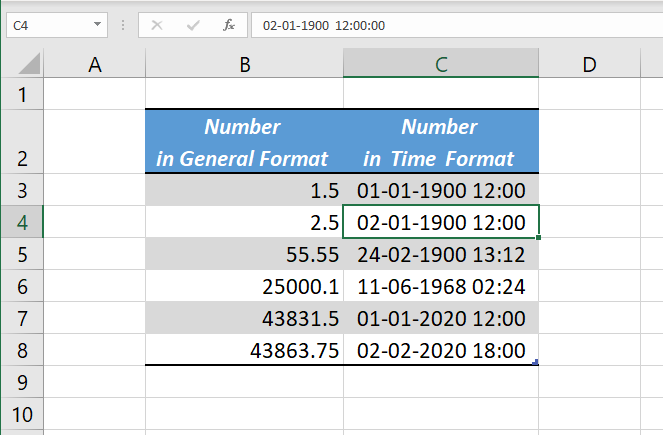# Date and Time in ExcelExcel treats Date and Time as numbers. In other words, Date and Time are stored as numbers in Excel. It is the Formatting of cells, which decides whether the content is to be displayed as a number or Date or Time.

#### Dates in Excel

Dates in Excel are stored as positive Integers starting from number 1.

Serial number 1 is equal to the date January 1, 1900.

Serial number 2 is equal to the date January 2, 1900, Serial number 25000 is 11-06-1968, Serial number 43863 is 02-02-2020 and so on

#### Time in Excel

Similarly, Excel store Times as decimals between 0 and 1 which represents fractions of a day.

0 is equal to 00:00:00 hours

1 Hour = 1/24 (1 Day/24 hours)

1 Minute = 1/(24×60) (1 Day/24 hours x 60 minutes)

1 Second = 1/(24×60) (1 Day/24 hours x 60 minutes x 60 seconds)

Similarily,

0.25 = 06:00:00 hours

0.75 = 18:00:00 hours

0.99 = 23:45:36 hours

#### Serial numbers representing a specific Time on a particular Date

As integers represent date and decimals represent time, the combination these two can represent a specific time on a specific date.

For example the number 1.5. The integer part 1 will represent the date January 1, 1900 and the decimal part 0.5 represent the time 12:00:00

1.5 = 01-01-1900 12:00:00

TODAY Function

UNIQUE function

SORT function

SEQUENCE function

SORTBY function

RANDARRAY function

This site uses Akismet to reduce spam. Learn how your comment data is processed.